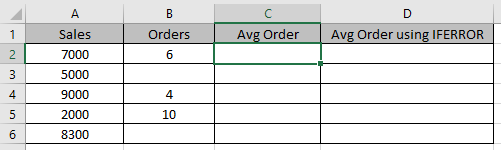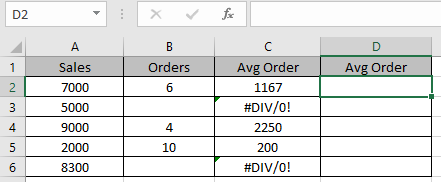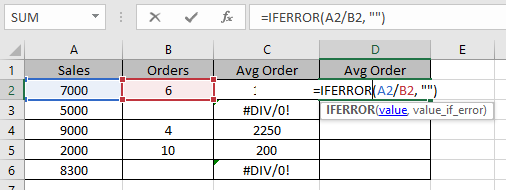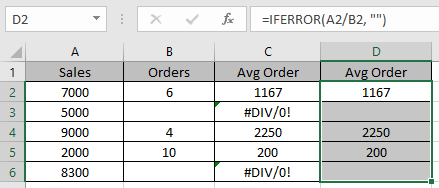# How to Use IFERROR Function in Excel

In this article, we will learn how to use IFERROR function to ignore the #N/A error while calculating the formula in Excel.
IFERROR function runs the logic_test in Excel. It catches error in a formula and returns an alternative result or formula when an error is detected. #N/A, #VALUE!, #REF!, #DIV/0!, #NUM!, #NAME?, and #NULL! These errors are checked under IFERROR.
Syntax:

=IFERROR (value, value_if_error)

Let’s learn this function using it in an example.
Here we have some dataUse the formula in C2 cell.

=A2/B2

Copy the formula in other cells, select the cells taking the first cell where the formula is already applied, use shortcut key Ctrl + DAs you can see it generates division error here.
Use the IFERROR formula

=IFERROR(A2/B2, “”)

This formula returns blank cell if error is generated in the division operator else it returns the value after division.Press Enter and Copy the formula in other cells, select the cells taking the first cell where the formula is already applied, use shortcut key Ctrl + DThat’s the difference it makes.
Hope you understood How to use IFERROR function to ignore errors. Explore more articles here on logical test. Please state your queries in the comment box below.

Related Articles:

How to Trace and Fix Formula Errors in Excel

Use VLOOKUP from Two or More Lookup Tables

Popular Articles:

50 Excel Shortcuts to Increase Your Productivity

How to use the VLOOKUP Function in Excel

How to use the COUNTIF function in Excel 2016

How to Use SUMIF Function in Excel

Terms and Conditions of use

The applications/code on this site are distributed as is and without warranties or liability. In no event shall the owner of the copyrights, or the authors of the applications/code be liable for any loss of profit, any problems or any damage resulting from the use or evaluation of the applications/code.